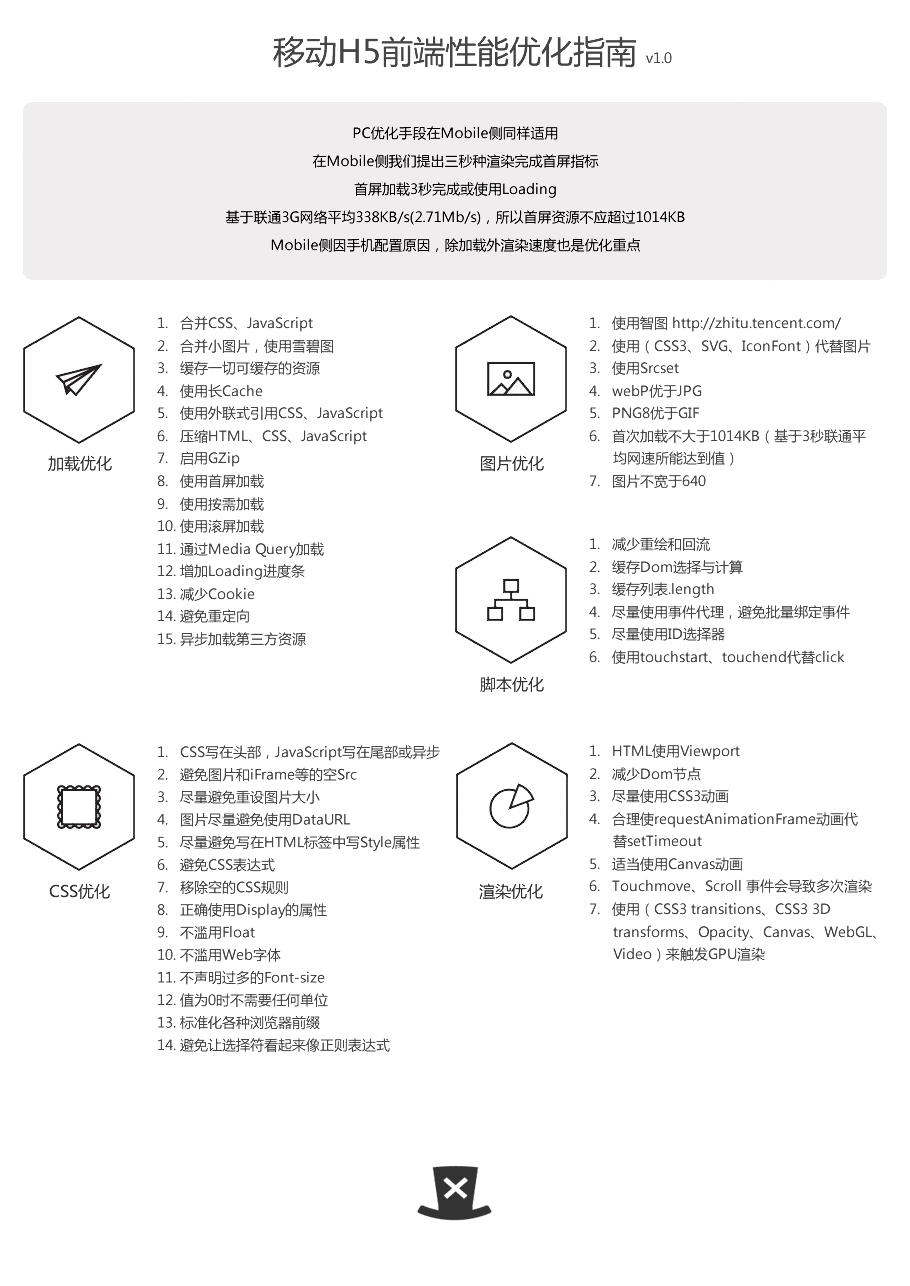jquery性能最佳实践

jquery
for访length
var myLength = myArray.length;
for (var i = 0; i < myLength; i++) {
// 要做的事
}

DOMDOM
// 别这样
$.each(reallyLongArray, function(count, item) { var newLI = '<li>' + item + '</li>';$('#ballers').append(newLI);
});
//较好的做法
var frag = document.createDocumentFragment();
$.each(reallyLongArray, function(count, item) { var newLI = '<li>' + item + '</li>'; frag.appendChild(newLI); });$('#ballers').appendChild(frag);each()$('#id')domdocument.createDocumentFragment()DOM // 或者这样 var myHtml = '';$.each(myArray, function(i, item) {
html += '<li>' + item + '</li>';
});
$('#ballers').html(myHtml); // 不理想 if ($ventfade.data('currently') != 'showing') {
$ventfade.stop(); } if ($venthover.data('currently') != 'showing') {
$venthover.stop(); } if ($spans.data('currently') != 'showing') {
$spans.stop(); } // 较好的 var elems = [$ventfade, $venthover,$spans];
$.each(elems, function(k, v) { if (v.data('currently') != 'showing') { v.stop(); } }) 使 // 不要这样$(document).ready(function() {...
$('#magic').click(function(e) {$('#yayeffects').slideUp(function() {...
});
});
$('#happiness').load(url + ' #unicorns', function() {... }) }); // 较好的 var PI = { onReady: function() {...$('#magic').click(PI.candyMtn);
$('#happiness').load(url + ' #unicorns', PI.unicornCb); }, candyMtn: function(e) {$('#yayeffects').slideUp(PI.slideCb);
},
slideCb: function() {...
},
unicornCb: function() {...
}
}
$(document).ready(PI.onReady); DOM , ID使#idelement // 非常快$('#container div.robotarm');
// 超级快
$('#container').find('div.robotarm');使$.fn.findjqueryID.JavascriptgetElementById()DOMID

// 未优化
$('div.data .gonzalez'); // 优化后$('.data td.gonzalez');使tag.classtag.class

$('.data table.attendees td.gonzalez'); // 不写中间的会更好$('.data td.gonzalez');DOM

$('.buttons > *'); // 极慢$('.buttons').children(); // 快很多
$('.gender :radio'); // 无定向搜索$('.gender *:radio'); // 同上
$('.gender input:radio'); // 这样好很多 使 jQuery$.fn.live$.fn.delegate使$.fn.delegate$.fn.live$.fn.live80 % 使

// 不好的 (如果列表里面元素很多)
$('li.trigger').click(handlerFn); // 较好的: event delegation with$.fn.live
$('li.trigger').live('click', handlerFn); // 最优的:$.fn.delegate
$('#myList').delegate('li.trigger', 'click', handlerFn); 移除元 DOMjQuery1.4$.fn.detachDOM
var $table =$('#myTable');
var $parent = table.parent();$table.detach();
// ... 添加大量的行到表格中
$parent.append(table); . detach().remove(), .detach()jQueryDOM CSS使$.fn.css20CSSstyle60 %
// 多于20明显慢
$('a.swedberg').css('color', '#asd123');$('<style type="text/css">a.swedberg { color : #asd123 }</style>').appendTo('head');

// 常用
$(elem).data(key, value); // 快十倍$.data(elem, key, value);

jQuery
//太遭了，执行了三个方法后才意识到里面是空的
$('#nosuchthing').slideUp(); // 较好 var$mySelection = $('#nosuchthing'); if ($mySelection.length) {
mySelection.slideUp();
}

// 最佳: add a doOnce plugin
jQuery.fn.doOnce = function(func) {
this.length && func.apply(this);
return this;
}
$('li.cartitems').doOnce(function() { // make it ajax! \o/ }); jQuery UI widget使 // 老套写法 var test = 1; var test2 = function() {... }; var test3 = test2(test); // var test = 1, test2 = function() {... }, test3 = test2(test);(function(foo, bar) {... })( 1, 2); // 旧方法 if (type == 'foo' || type == 'bar') {... } // 好方法 if (/^(foo|bar)$/.test(type)) {...
}

// 查找对象
if (({
foo: 1,
bar: 1
})[
type]) {...

}posted @ 2010-12-05 19:00  曾祥展  阅读(1313)  评论(6编辑  收藏  举报